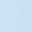Discussion about math, puzzles, games and fun.   Useful symbols: ÷ × ½ √ ∞ ≠ ≤ ≥ ≈ ⇒ ± ∈ Δ θ ∴ ∑ ∫  π  -¹ ² ³ °

You are not logged in.

## #1 2019-12-17 14:19:20

666 bro
MemberFrom: Flatland
Registered: 2019-04-26
Posts: 586

### P(aub) =p(a) +p(b) -p(a&b)

In order to consistently define the values of p(a) and p(b)  why p(a&b)
≤ p(a) and p(b)?

"An equation for me has no meaning, unless it expresses a thought of God"- Srinivasa ramanujan

Offline

## #2 2019-12-17 22:08:18

BobRegistered: 2010-06-20
Posts: 8,914

### Re: P(aub) =p(a) +p(b) -p(a&b)

hi 666 bro

I'm not quite sure what you're asking so here's some general help with this sort of probability.  I'm re-using an old diagram to save making a fresh one as it will work for this.  Let's say that a=bread and b=milk.There are 100 houses in an area.  Some have bread delivered; some have milk; some have both; and some have neither.  If you pick a house at random what's the probability of …..

p(a) ie. probability of picking a house that has bread delivered = 60/100

p(b) = ie. the probablilty of picking a house that has milk delivered = 50/100

p(a OR b) = ie. the probability of picking a house that has either bread or milk or both = 80/100

p(a AND b) = ie. the probability of picking a house that has both delivered = 30/100

You can see that p(a OR b) = p(a) + p(b) - p(a AND b) [80/100 = 60/100 + 50/100 - 30/100]

This will always be true because when you add p(a) to p(b) you get all of a, all of b but you count (a AND b) twice.

As (a AND b) is contained in both a and b it will never be greater than their sum.  It could be equal if, say, the region of b that doesn't overlap a is empty.

Bob

Children are not defined by school ...........The Fonz
You cannot teach a man anything;  you can only help him find it within himself..........Galileo Galilei
Sometimes I deliberately make mistakes, just to test you!  …………….BobOffline

## #3 2019-12-17 23:07:31

666 bro
MemberFrom: Flatland
Registered: 2019-04-26
Posts: 586

### Re: P(aub) =p(a) +p(b) -p(a&b)

I'm asking   what are the general conditions for defining the  existing value of p(a&b)?

"An equation for me has no meaning, unless it expresses a thought of God"- Srinivasa ramanujan

Offline

## #4 2019-12-18 00:21:49

BobRegistered: 2010-06-20
Posts: 8,914

### Re: P(aub) =p(a) +p(b) -p(a&b)

I'm still unclear.

a must be an event for which a probability can be estimated or determined.

b similarly.

In that case a & b exists as a measurable event.  It means both a and b happen.

Bob

Children are not defined by school ...........The Fonz
You cannot teach a man anything;  you can only help him find it within himself..........Galileo Galilei
Sometimes I deliberately make mistakes, just to test you!  …………….BobOffline

## #5 2020-02-01 01:36:11

George,Y
MemberRegistered: 2006-03-12
Posts: 1,379

### Re: P(aub) =p(a) +p(b) -p(a&b)

The logical definition of A&B is that both of them are true.

Thus from a logical point of view, !A and A cannot be true simultaneously. This is by definition of logic.

also !A+A = ALL

B = (!A U A)&B =  ( !A&B )U (A&B)

The first equal sign is self-explanatory, here is the rational for the second:

If a case belongs to !A&B, it must belong to (!A U A)&B since !A U A contains !A
same for A&B
Therefore the left contains the right

you can then prove the right contains the left from a similar logic.

Therefore
B contains A&B.

Last edited by George,Y (2020-02-01 01:36:26)

X'(y-Xβ)=0

Offline